• python容器之元组 1. 元组的创建： #直接创建 t = (1,2) #使用tuple(): t = tuple((1,2)) 2.元组在内存单元中是有序的，可以通过下标访问，且下标可以为负数 t = tuple((1,2,3)) #输出元组t所有元素 for i in range...
python容器之元组
1. 元组的创建：
#直接创建
t = (1,2)
#使用tuple():
t = tuple((1,2))
2.元组在内存单元中是有序的，可以通过下标访问，且下标可以为负数
t = tuple((1,2,3))
#输出元组t所有元素
for i in range(3)：
print(t[i])
i += 1
#输出元组t中的元素3
print(t[-1])		
3.元组常用方法：
count()  计算元组中元素出现的次数
index() 访问元组元素的下标
4. 元组中可以存在列表、集合等不同数据类型：
t = tuple((1,{1},))


展开全文• # 元组(tuple)是 Python 中另一个重要的序列结构，和列表类似，元组也是由一系列按特定顺序排序的元素组成。元组是一个有序列表，序列中的每个元素都分配一个数字(位置，索引)# 1、我们可以使用圆括号，小括号()来...


# 元组(tuple)是 Python 中另一个重要的序列结构，和列表类似，元组也是由一系列按特定顺序排序的元素组成。元组是一个有序列表，序列中的每个元素都分配一个数字(位置，索引)# 1、我们可以使用圆括号，小括号()来创建元组# 2、我们可以直接将序列放在tuple(seq)#元组中的元素支持python中任何数据类型，个数没有限制tup = (3,'v',['a',1,2],(1,2,3,4,5),{'a':11,'b':13})print(tup)print(type(tup))# 创建一个元组,两种创建方式a = (1,2,3,4,5,6,7)b = tuple("abcdefg")print(a)print(type(a))print(b)print(type(b))# 创建一个空的元组c= ()d = tuple()print(c)print(type(c))print(d)print(type(d))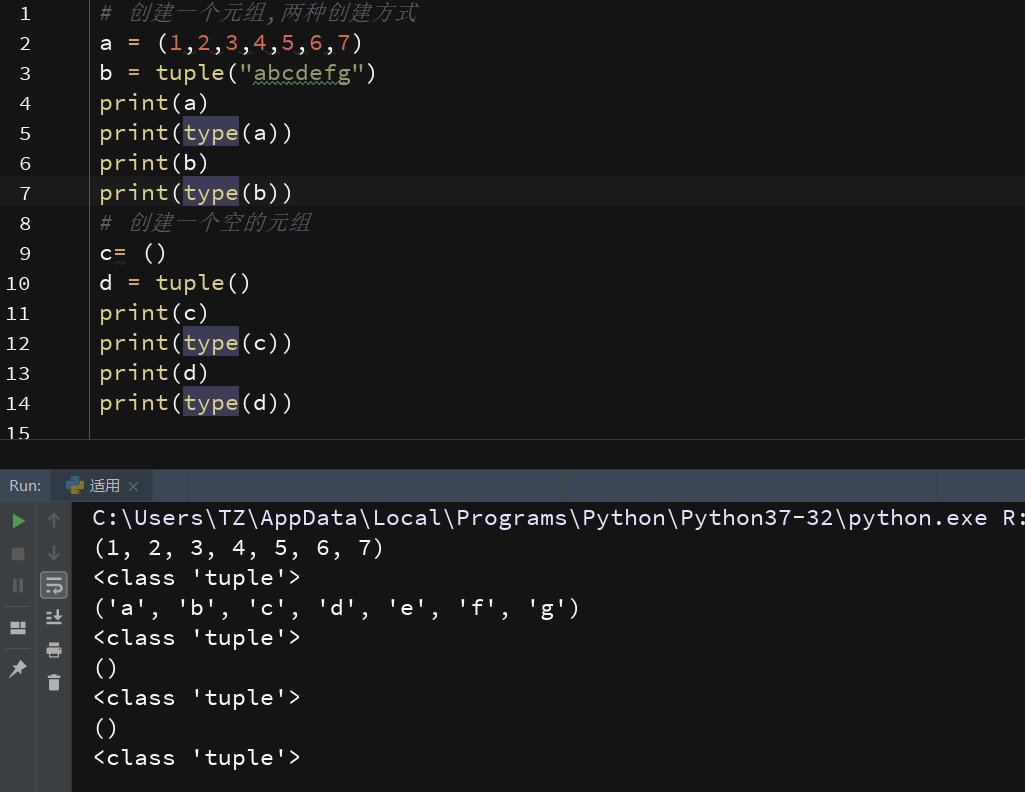# 元组中只包含一个元素时，需要在元素后面添加逗号，否则括号会被当作运算符使用：e = (10)print(e)print(type(e))f = (10,)print(f)print(type(f))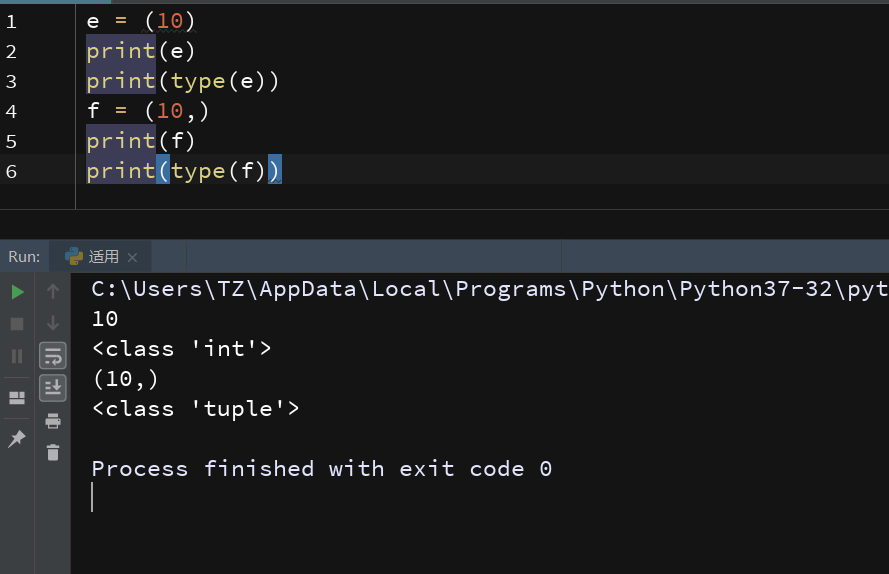# 元组是有序的序列，存在下标index，索引从0开始# 元组同样支持负数，负数从-1开始# Python中访问元组与列表一样，通过索引print(a)print(a[-1])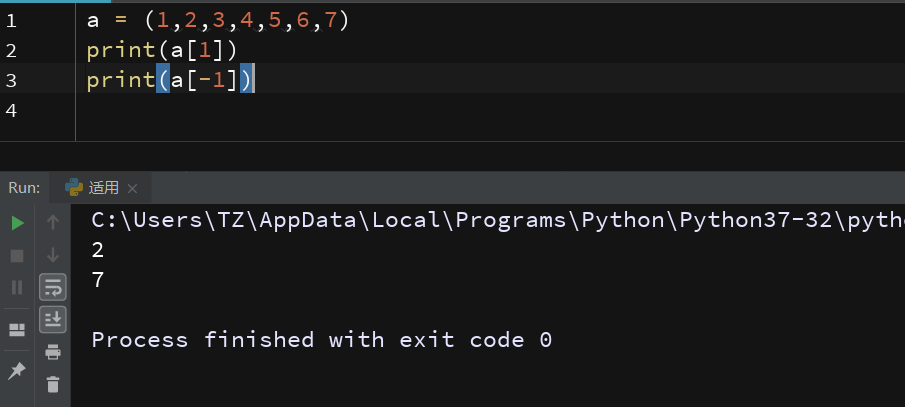# 元组的常用操作# 元组的修改# 元组的删除# 元组的组合# 元组的重复# 元组与列表的区别：元组的元素不可修改 ，列表可修改# 元组是不可变序列，列表是可变序列# a = a# print(a)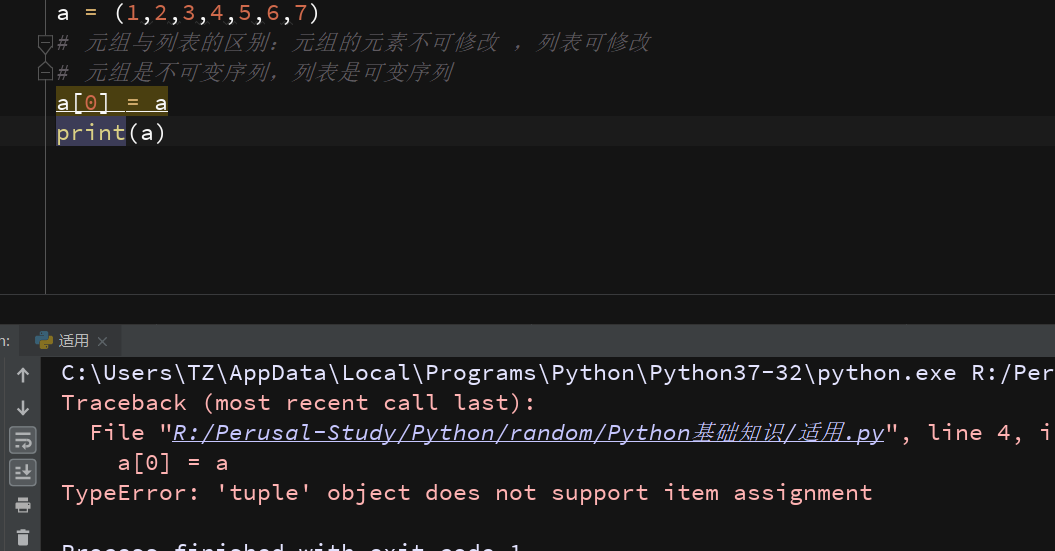# 元组中的元素不可删除，但可以使用del删除整个元组# 删除单个元素# del a# 删除整个元组后，输出元组会报错# del a# print(a)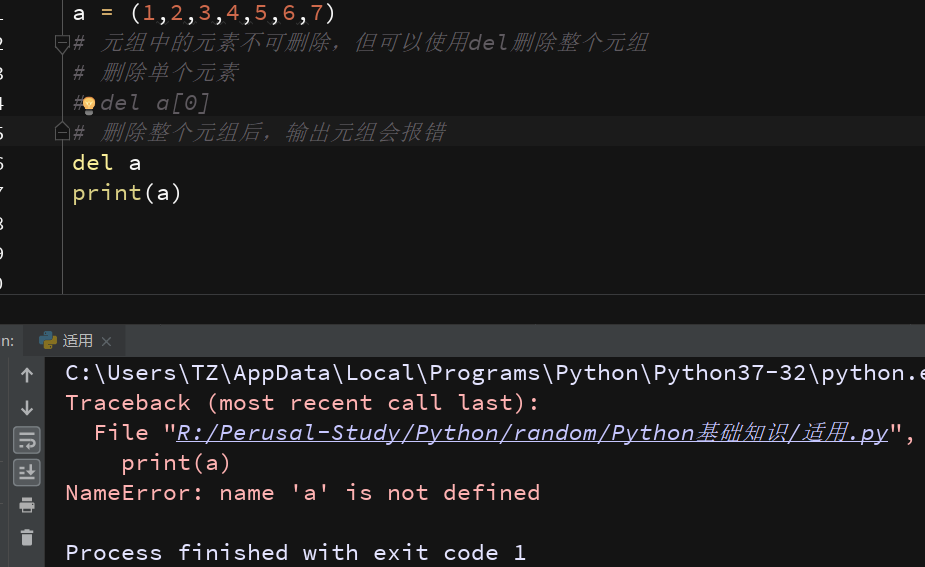# 可重复可组合print(a + b)print(a*3)# 通过连接两个元组，创建一个新的元组a1 = a + bprint(a1)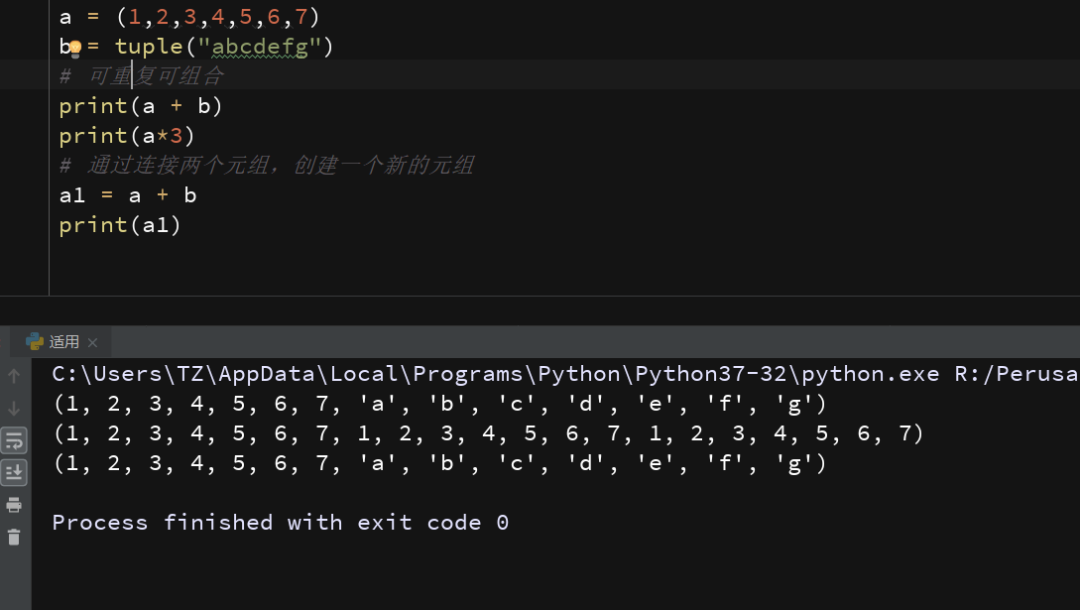# 元组常用方法# len()# max()# min()# 获取元组的元素个数print(len(a))# 获取元组中最大值print(max(a))# 获取元组中最小值print(min(a))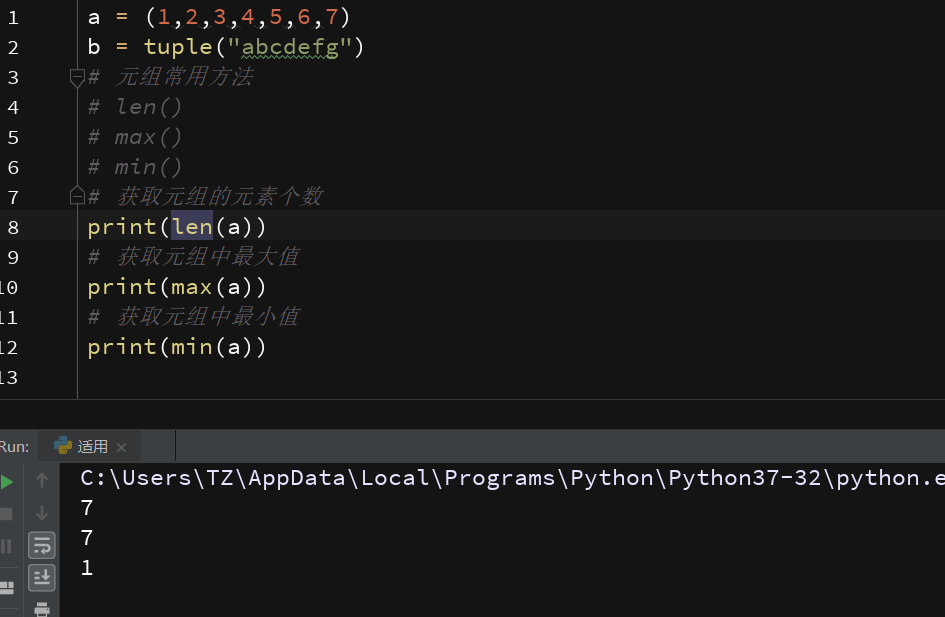# 字符串、列表、元组、字典、区间的转换a3 = ("a","b","c")# 元组转列表a5= list(a3)print(a5)print(type(a5))# 列表转元组a6= tuple(a5)print(a6)# 字典转元组,元组无法转换为字典a7 = {"你":"我","她":"他","he":"she"}a8= tuple(a7)print(type(a7))print(a8)print(type(a8))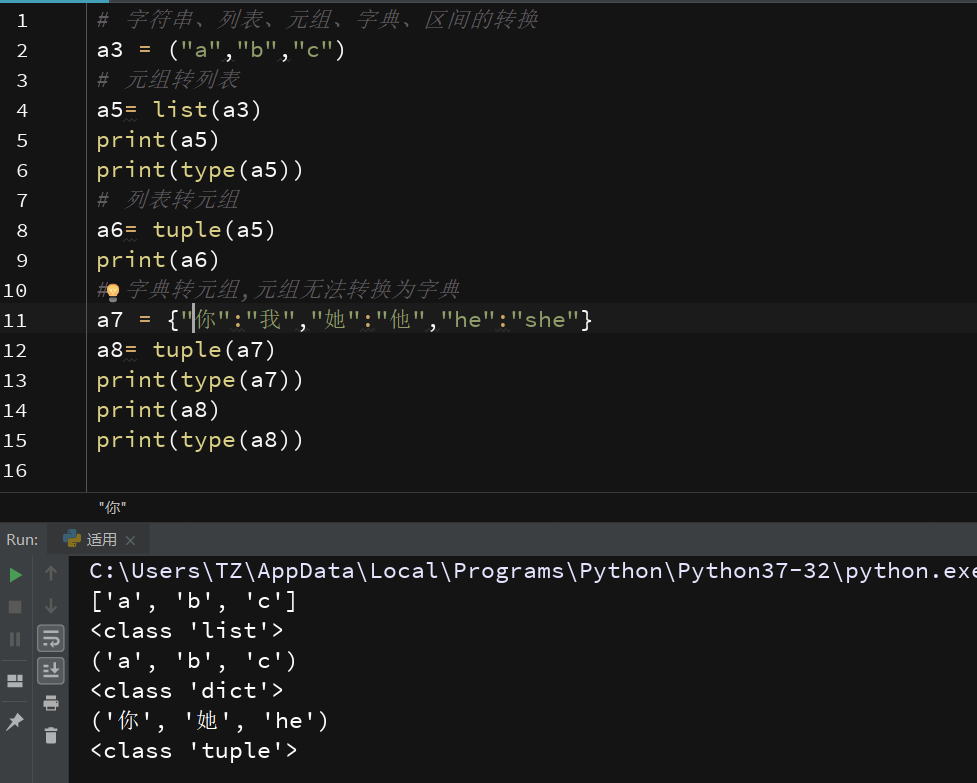# 元组转字符串a9= str(a3)print(a9)print(type(a9))# 字符串转元组a10= tuple(a9)print(a10)# 区间转元组，元组不能转换为区间a11 = range(1,10)a12= tuple(a11)print(a12)print(type(a12))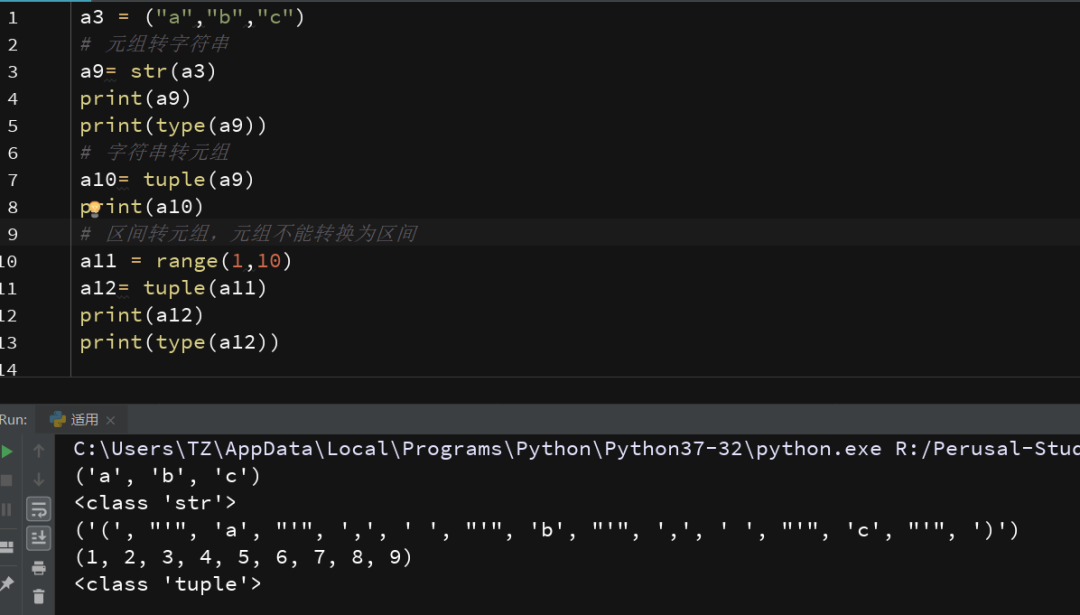展开全文• 我在python中开发了一个文件类，它可以读写一个文件，包含一个xyz坐标列表。在我的程序中，我已经有一个Coord3D类来保存xyz坐标。我的问题是关于getCoordinate(index)方法的设计。我应该返回一个float元组，还是一个...
我在python中开发了一个文件类，它可以读写一个文件，包含一个xyz坐标列表。在我的程序中，我已经有一个Coord3D类来保存xyz坐标。我的问题是关于getCoordinate(index)方法的设计。我应该返回一个float元组，还是一个Coord3D对象？在第一种情况下，耦合度很低，但无论如何，我可能必须用获得的值实例化Coord3D对象，尽管不在file类中。在第二种情况下，我将文件类与Coord3D类紧密耦合。请注意，我认为这两种解决方案没有太大的区别，但我想看看你的答案和背后的原因。编辑：要概括我到目前为止得到的答案，似乎没有明确的选择。有人说(恰当地说)python不是Java，您不需要专门的类来处理所有事情，因为您需要按语言体系结构进行处理。但就我而言，我有以下条件：我正在一个库中工作，其中Coord3D对象按原样使用。使用它将增加我的库的内聚性，因为数据类型将被统一使用。Coord3D对象具有状态和行为。实际上，Coord3D对象将坐标和单位聚合在一个实体中。Coord3D对象之间的操作将考虑到潜在的不同单元，并相应地采取行动。我可以将集中控制代码放入Coord3D类实例化中以拒绝，例如，长度为4的数组或非单元数组。如果使用元组，则无法执行此检查。此外，如果一个方法接受Coord3D，则可以保证它是格式良好的预先(您可以使用粗体并检查isinstance或检查接口)。元组可以包含无效数据。尽管python的错误处理方法是在出现问题的地方完成的，但是一个阻止我使用由三个字符串组成的xyz坐标的类在某种程度上是有益的(如果错误，请纠正我)另一方面，使用元组具有以下优点：占用资源少，在巨大的更简单的设计。更多的类意味着更复杂的设计。元组是一种标准的数据类型，它很容易理解和解包。个性化的类不是。使用元组时，XYZFile类与库的其余部分完全分离(因为它不使用Coord3D对象)。这意味着它可以作为一个独立的实体完全重用。欢迎进一步评论！
展开全文• I've written a program that gives me a list of tuples. I need to grab the tuple with with the max number in the second value.(840, 32), (841, 3), (842, 4), (843, 4), (844, 6), (845, 6), (846, 12), (84...I've written a program that gives me a list of tuples. I need to grab the tuple with with the max number in the second value.(840, 32), (841, 3), (842, 4), (843, 4), (844, 6), (845, 6), (846, 12), (847, 6), (848, 10), (849, 4), ..snip...I need to get back (840,32) because 32 is the highest second number in the tuple. How can I achieve this? I've tried a variety of ways but keep getting stuck here is the complete code:D = {}def divisor(n):global DL = []for i in range(1,n+1):if n % i == 0:L.append(i)D[n] = len(L)for j in range(1001):divisor(j)print(D.items())解决方案Use max() with lambda:In : lis=[(840, 32), (841, 3), (842, 4), (843, 4), (844, 6), (845, 6), (846, 12), (847, 6), (848, 10), (849, 4)]In : max(lis, key=lambda x:x)Out: (840, 32)In : import operatorIn : max(lis, key=operator.itemgetter(1))Out: (840, 32)
展开全文• Python 元组Python的元组与列表类似，不同之处在于元组的元素不能修改。元组使用小括号，列表使用方括号。元组创建很简单，只需要在括号中添加元素，并使用逗号隔开即可。如下实例：实例(Python 2.0+)tup1=('physics...
• 摘要：在python中，有一种数据类型，它的成员是有序排列的，可以通过下标偏移量访问，称之为“序列”，包括字符串、列表、元组。我们主要从简介、操作符、内建函数、特性、相关模块等方面来学习这些序列。 本文...字符串
• python元组里嵌套元组 Python元组 (Python Tuples) Tuples in Python are a collection of elements in a round bracket() or not but separated by commas. Tuples are similar to list in some operations like ...
• Python 元组Python的元组与列表类似，不同之处在于元组的元素不能修改。元组使用小括号，列表使用方括号。元组创建很简单，只需要在括号中添加元素，并使用逗号隔开即可。如下实例：tup1 = ('physics', 'chemistry',...
• Python 元组Python的元组与列表类似，不同之处在于元组的元素不能修改。元组使用小括号，列表使用方括号。元组创建很简单，只需要在括号中添加元素，并使用逗号隔开即可。如下实例：tup1 = ('physics', 'chemistry',...
• python创建空元组Python | 空元组 (Python | empty tuple)In python, we can also create a tuple without having any element. An empty tuple is created using a pair of round brackets, ().在python中，我们也...
• Python 元组Python的元组与列表类似，不同之处在于元组的元素不能修改。元组使用小括号，列表使用方括号。元组创建很简单，只需要在括号中添加元素，并使用逗号隔开即可。如下实例：实例(Python 2.0+)tup1 = ('...
• Python 元组Python的元组与列表类似，不同之处在于元组的元素不能修改。元组使用小括号，列表使用方括号。元组创建很简单，只需要在括号中添加元素，并使用逗号隔开即可。创建空元组tup1=();元组中只包含一个元素时...
• Python 元组 Python元组与列表类似，不同之处在于元组的元素不能修改。 元组使用小括号，列表使用方括号。 元组创建很简单，只需要在括号中添加元素，并使用逗号隔开即可。 1.创建空元组 2.访问元组 元组可以使用...
• 文章目录Python创建元组1) 使用 ( ) 直接创建2) 使用tuple()函数创建元组Python访问元组元素Python修改元组Python删除元组Python元组和列表的区别 元组（tuple）是 Python 中另一个重要的序列结构，和列表类似，元组...
• 文章首发微信公众号，微信搜索：猿说python在上一篇文章中我们讲解了关于python列表List的相关内容，今天给大家解释一下列表List的兄弟 – 元组，俗称: tuple.元组tuple和列表List类似，元组有如下特点：1.由一个...
• python创建空元组 Python | 空元组 (Python | empty tuple) In python, we can also create a tuple without having any element. An empty tuple is created using a pair of round brackets, (). 在python中，...列表 anaconda 深度学习
• Python元组和列表类似，不同之处在于元组中的元素不能修改（因此元组又称为只读列表），元组使用原括号括起。1、元组中只包含一个元素时，需要在元素后边添加一个逗号消除歧义。2、元组中的值是不允许修改的，...
• 元组 Python元组与列表类似，不同之处在于元组的元素不能修改。元组使用小括号，列表使用方括号。 1.访问元组 无法修改元组 index 类型转换 字典
• Python 元组Python的元组与列表类似，不同之处在于元组的元素不能修改。元组使用小括号，列表使用方括号。元组创建很简单，只需要在括号中添加元素，并使用逗号隔开即可。如下实例：实例(Python 2.0+)tup1=('physics...
• PythonPython 元组删除元组元组运算符元组索引，截取无关闭分隔符元组内置函数 Python 元组 Python元组与列表类似，不同之处在于元组的元素不能修改。 元组使用小括号，列表使用方括号。 元组创建很简单，只需要在...
• Python元组与列表相似，不同之处在于元组的元素不能修改元组使用小括号，列表使用方括号元组创建很简单，只需要在括号中添加元素，并使用逗号隔开即可。创建空元组tup1 =();tup2= (1,)元组只包含一个元素时，需要...
• Python元组(tuple)与列表类似，不同之处在于元组的元素不能修改，元组使用圆括号包含元素，而列表使用方括号包含元素。元组的创建很简单，只需要在圆括号中添加元素，并使用逗号分隔即可，示例代码如下：tuple_one...
• python 初始化 元组 Python | 重新初始化元组 (Python | Reinitializing tuple) In this tutorial, we will learn how can we reinitialize a tuple with a new set of elements/objects? 在本教程中，我们将学习...深度学习 机器学习 列表 编程语言...

# 元组pythonpython 订阅